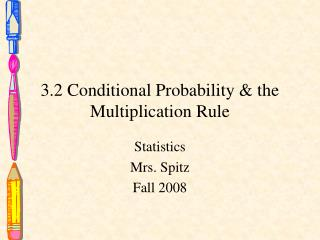DownloadDownload Presentation3.2 Conditional Probability & the Multiplication Rule

# 3.2 Conditional Probability & the Multiplication Rule

Télécharger la présentation## 3.2 Conditional Probability & the Multiplication Rule

- - - - - - - - - - - - - - - - - - - - - - - - - - - E N D - - - - - - - - - - - - - - - - - - - - - - - - - - -
##### Presentation Transcript

1. 3.2 Conditional Probability & the Multiplication Rule Statistics Mrs. Spitz Fall 2008

2. Objectives/Assignment • How to find the probability of an even given that another event has occurred. • How to distinguish been independent and dependant events. • How to use the multiplication rule to find the probability of two events occurring in a sequence • How to use the multiplication rule to find conditional probabilities. • Assignment: pp. 119-122 #1-20

3. Conditional Probability • Is the probability of an event occurring given that another event has already occurred. The conditional probability of event B occurring, given that event A has occurred, is denoted by P(B|A) and is read as “probability of B, given A.”

4. Finding conditional probabilities • The table shows the results of a study in which researchers examined a child’s IQ and the presence of a specific gene in the child. Find the probability that the child has a high IQ, given that the child has the gene. Solution: There are 72 children who have the gene. So, the sample space consists of these 72 children. Of these, 33 have high IQ. So, P(B|A) = 33/72 ≈ .458

5. Independent and Dependent Events • The question of the interdependence of two or more events is important to researchers in fields such as marketing, medicine, and psychology. You can use conditional probabilities to determine whether events are independent or dependent.

6. Definition • Two events are independent if the occurrence of one of the events does NOT affect the probability of the occurrence of the other event. Two events A and B are independent if: P(B|A) = P(B) or if P(A|B) = P(A) Events that are not independent are dependent.

7. Classifying events as independent or dependent • Decide whether the events are independent or dependent. 1. Selecting a king from a standard deck (A), not replacing it and then selecting a queen from the deck (B). Solution: P(B|A) = 4/51 and P(B) = 4/52. The occurrence of A changes the probability of the occurrence of B, so the events are dependent.

8. Classifying events as independent or dependent • Decide whether the events are independent or dependent. 2. Tossing a coin and getting a head (A), and then rolling a six-sided die and obtaining a 6 (B). Solution: P(B|A) = 1/6 and P(B) = 1/6. The occurrence of A does not change the probability of the occurrence of B, so the events are independent.

9. Classifying events as independent or dependent • Decide whether the events are independent or dependent. 3. Practicing the piano (A), and then becoming a concert pianist (B). Solution: If you practice the piano, the chances of becoming a concert pianist are greatly increased, so these events are dependent.

10. The Multiplication Rule • To find the probability of two events occurring in a sequence, you can use the multiplication rule. The probability that two events A and B will occur in sequence is P(A and B) = P(A) ● P(B) If events A and B are independent, then the rule can be simplified to P(A and B) = P(A) ● P(B). This simplified rule can be extended for any number of events.

11. Using the multiplication rule to find probabilities Two cards are selected without replacement, from a standard deck. Find the probability of selecting a king and then selecting a queen. Solution: Because the first card is not replaced, the events are dependent. P(K and Q) = P(K) ● P(Q|K) So the probability of selecting a king and then a queen is about .0006

12. Using the multiplication rule to find probabilities A coin is tossed and a die is rolled. Find the probability of getting a head and then rolling a 6. Solution: The events are independent P(H and 6) = P(H) ● P(6) So the probability of tossing a head and then rolling a 6 is about .0083

13. Note: • To determine if A and B are independent, calculate P(B) and P(B|A). If the values are equal, then the events are independent and the multiplication rule is simplified.

14. Using the multiplication rule to find probabilities • A coin is tossed and a die is rolled. Find the probability of getting a head and then rolling a 2. P(H) = ½. Whether or not the coin is a head, P(2) = 1/6—The events are independent. So, the probability of tossing a head and then rolling a two is about .083.

15. Using the multiplication rule to find probabilities • The probability that a salmon swims successfully through a dam is .85. Find the probability that 3 salmon swim successfully through the dam. The probability that each salmon is successful is .85. One salmon’s chance of success is independent of the others. So, the probability that all 3 are successful is about .614.

16. Using the multiplication rule to find probabilities • Find the probability that none of the salmon is successful. So, the probability that none of the 3 are successful is about .003.

17. Using the multiplication rule to find probabilities • Find the probability that at least one of the salmon is successful in swimming through the dam. So, the probability that at least one of the 3 are successful is about .997.